# Example: Using DistArray for Gaussian Elimination (L-U decomposition)¶

Note: This notebook requires an IPython.parallel cluster to be running. Outside the notebook, run:

dacluster start -n4

Gaussian Elimination is an algorithm in Linear Algebra best understood as a series of row operations on the coefficient matrix of a system of linear equations. The method can also be used to calculate the rank, determinant, and inverse of a matrix. The algorithm involves adding multiples of each row to subsequent rows, in order to make the coefficient matrix upper triangular. The resulting system is then solved by back-substitution which is trivial to perform. This algorithm is illustrated in the figure below:Here i refers to the index of the pivot row (started at 1). Please note that much of this example notebook is adapted from Parallel Gaussian Elimination which is a good starting point for gaining a better understanding of Gaussian Elimination from an computational standpoint.

In this notebook we will demonstrate how to perform GE in parallel using the DistArray API. The main challenge in parallelizing Gaussian Elimination on a distributed memory machine is that the calculation of each row requires the calculation of all rows that have come before it and therefore concurrency of operations becomes the overriding issue. This bodes well for the client-engine architecture of IPython.parallel (and consequently DistArray) as the client can keep track of pivot row, while the row transformations can be pushed out to the engines using custom uFuncs.

We begin with the imports:

In :
# utility imports
from __future__ import print_function
from pprint import pprint
from matplotlib import pyplot as plt

# main imports
import numpy as np
import distarray.globalapi as da
from distarray.plotting import plot_array_distribution

# output goodness
np.set_printoptions(precision=2)

# display figures inline
%matplotlib inline


We now define the parameter space for our study. We will perform GE on matrices that are block distributed in any one or both dimensions, while simultaneously varying the size:

In :
distributions = [('n','b'), ('b','n'), ('b','b')]
sizes = [8, 16, 32, 64]
print(distributions)

[('n', 'b'), ('b', 'n'), ('b', 'b')]


Next, we create a context and devise a scheme for generating some synthetic data (in this case a matrix) on which to operate:

In :
context = da.Context()
def synthetic_data_generator(contextobj, datashape=(16, 16), distscheme=('b', 'n')):
"""Return objective matrix with specified size and distribution."""
distribution = da.Distribution(contextobj, shape=datashape, dist=distscheme)
_syndata = np.random.random(datashape)
syndata = contextobj.fromarray(_syndata, distribution=distribution)
return syndata


In order for the Gaussian Elimination operation to be truly parallel, we need to define a uFunc to perform the desired computation:

In :
def parallel_gauss_elim(darray, pivot_row, k, m):
"""
Perform in-place gaussian elimination locally on all engines.

Parameters
---------
darray : DistArray
Handle for the array to be manipulated (global)
pivot_row : numpy.ndarray
Array containing pivot row (global)
k : integer
Pivot row index (global)
m : numpy.ndarray
Vector containing pivoting factors (global)
"""
import numpy as np

# retrieve local indices for submatrix that needs to be operated on
n_rows, n_cols = darray.distribution.global_shape
i_slice, j_slice = darray.distribution.local_from_global((slice(k+1, n_rows),
slice(k, n_cols)))

# limit the slices using actual size of local array
n_rows_local, n_cols_local = darray.ndarray.shape
i_indices, j_indices = (i_slice.indices(n_rows_local),
j_slice.indices(n_cols_local))

# determine which elements of global pivot row correspond to local entries
_, piv_slice = darray.distribution.global_from_local((slice(0, n_rows_local),
slice(*j_indices)))

# limit the slice to the size of the global pivot row
piv_indices = piv_slice.indices(n_cols)

# determine which elements of global pivot factor vector corresponds to local
mul_slice, _ = darray.distribution.global_from_local((slice(*i_indices),
slice(0, n_cols_local)))

# limit the slice to the size of the global pivot factor vector
mul_indices = mul_slice.indices(n_rows)

# perform the elimination to create zeros below pivot
if (i_indices == i_indices or j_indices == j_indices):
# computation for the local block is done
return
else:
for i, mul in zip(xrange(*i_indices), xrange(*mul_indices)):
np.subtract(darray.ndarray[i, slice(*j_indices)],
np.multiply(m[mul], pivot_row[slice(*piv_indices)]),
out=darray.ndarray[i, slice(*j_indices)])
return


We want to use a nice syntax for calling out uFunc hence we register it with our context (alternatively, we could have just used Context.apply which has a more obscure call format):

In :
context.register(parallel_gauss_elim)


All that is left now is to define the high level function that runs on the client and manages the GE operation. Using this function is a way of ensuring synchronicity between the many engines performing this operation. After a pivot row is determined, it is broadcast along with a vector of pivoting factors to the worker engines via the parallel_gauss_elim uFunc. Note how we have actually subverted the need to use canonical MPI constructs (in this case, MPI_Bcast()) by making use of the fact that our uFunc can accept arbitrary arguments.

In :
def execute_ge(contextobj, darray):
N = min(darray.shape)
for k in range(N-1):
pivot_factors = (d_array[:, k]/d_array[k, k]).toarray()
contextobj.parallel_gauss_elim(darray, darray[k, :].toarray(), k, pivot_factors)


In order to enable the reader to better visualize what is happening in this example, we will make the first set of runs with the size fixed at 8, while cycling through the distribution types. We also print out a graphical representation of the distribution of the resulting upper triangular matrices.

In :
N = sizes
for scheme in distributions:
d_array = 1000 * synthetic_data_generator(context, datashape=(N,N), distscheme=scheme)
execute_ge(context, d_array)
process_coords = [(0, 0), (1, 0), (2, 0), (3, 0)]
plot_array_distribution(d_array, process_coords, legend=True,
title=str("Distribution Scheme = " + str(scheme)))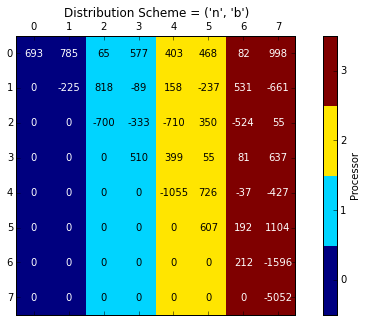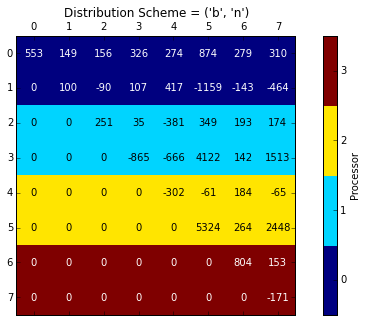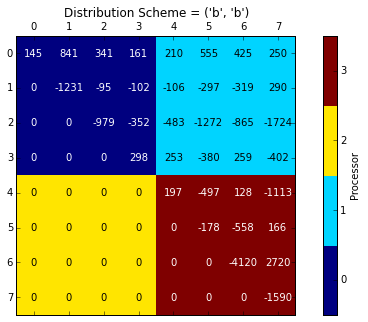Now we write a quick routine that runs through all sizes and distributions and records the runtimes. The resulting information is best represented as a plot the data for which is collected in a Dictionary called performance_data. Depending on the contents of your sizes vector, the runtimes may very a great deal on this section.

In :
# create a dictionary of lists
from collections import defaultdict
performance_data = defaultdict(list)

for scheme in distributions:
for N in sizes:
d_array = 1000 * synthetic_data_generator(context, datashape=(N,N), distscheme=scheme)
_time = %timeit -o execute_ge(context, d_array)
performance_data[scheme].append(_time.best)
plt.plot(sizes, performance_data[scheme], label=str(scheme), linewidth=2)

plt.legend(loc=4)
plt.xlabel('Problem Size N')
plt.ylabel('Execution Time [seconds]')
plt.title('Gaussian Elimination N vs t')
plt.grid(True)

1 loops, best of 3: 1.15 s per loop
1 loops, best of 3: 2.44 s per loop
1 loops, best of 3: 5.03 s per loop
1 loops, best of 3: 10.3 s per loop
1 loops, best of 3: 1.25 s per loop
1 loops, best of 3: 2.7 s per loop
1 loops, best of 3: 5.73 s per loop
1 loops, best of 3: 11.5 s per loop
1 loops, best of 3: 1.15 s per loop
1 loops, best of 3: 2.38 s per loop
1 loops, best of 3: 4.89 s per loop
1 loops, best of 3: 10.1 s per loop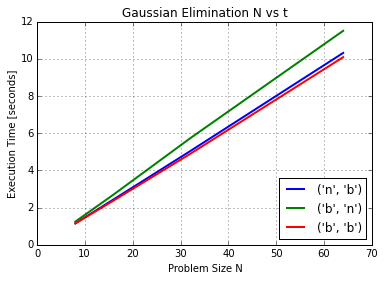We see that we observe similar performance from all three distributions with a block-block map marginally most efficient.

# Application: LU Decomposition¶

In this section we will demonstrate how we can, with minimal modification, use the GE approach to perform LU decomposition of a matrix.

Mathematically, LU-factorization follows naturally from GE operations on any given matrix (with the exception of systems that require partial pivoting - these need some special treatement and in this example we don't concern ourselves with these issues of numerical stability of GE). LU-factorization involves expressing a given matrix as a product of two matrices - one of which is lower triangular ($\mathbf{L}$), and the other upper triangular ($\mathbf{U}$):

$$\mathbf{A} = \mathbf{L} \mathbf{U}$$

Once we have this factorization, we can make use of it to solve $\mathbf{A}x=b$:

$$\mathbf{A}x = \mathbf{L}\mathbf{U}x = b$$

Which is equivalent to:

$$\mathbf{U}x = \mathbf{L}^{-1}b =:c$$

Since the cost of matrix inversion is prohibitively high, we find $c = \mathbf{L}^{-1} b$ through forward substitution:

$$\mathbf{L}c = b$$

This is easy to do as $\mathbf{L}$ is lower triangular. The final step then becomes to perform backward substitution:

$$\mathbf{U}x=c$$

The procedure demonstrated in the preceeding section gives us a method of finding $\mathbf{U}$, and now we are tasked with computing $\mathbf{L}$. If the series of elementary row operations that the GE process entails are expressed as a series of matrix transformations on $\mathbf{A}$ we end up with a series of trivially invertible matrices, which when multiplied give us $\mathbf{L}$:

$$\mathbf{E}_{n} \mathbf{E}_{n-1} ... \mathbf{E}_{2} \mathbf{E}_{1} \mathbf{A} = \mathbf{U}$$$$\implies \mathbf{A} = \mathbf{E}_{1}^{-1} \mathbf{E}_{2}^{-1} ... \mathbf{E}_{n-1}^{-1} \mathbf{E}_{n}^{-1} \mathbf{U}$$$$\implies \mathbf{E}_{1}^{-1} \mathbf{E}_{2}^{-1} ... \mathbf{E}_{n-1}^{-1} \mathbf{E}_{n}^{-1} =: \mathbf{L}$$

It turns out that $\mathbf{L}$ is basically composed of the negatives of the off-diagonal elements in the matrix transformations.

We now have enough information to write a routine to perform LU-decomposition. Our approach will be to perform the GE in-place on a copy of the objective matrix, and create a new matrix which will be populated with the multiplicative factors. The uFunc for GE will be used by us directly, we need only modify the driver function:

In :
def execute_lu(contextobj, darray):
# create placeholders for lower and upper triangular matrices
uarray = contextobj.fromarray(darray.toarray(), distribution=darray.distribution)
larray = contextobj.fromarray(np.zeros(darray.shape), distribution=darray.distribution)

N = min(darray.shape)
for k in range(N-1):
pivot_factors = (uarray[:, k]/uarray[k, k]).toarray()
pivot_factors[0:k] = 0.0
# populate lower triangular matrix
larray[:, k] = pivot_factors
contextobj.parallel_gauss_elim(uarray, uarray[k, :].toarray(), k, pivot_factors)
larray[-1, -1] = 1.0
return larray, uarray


Let us first test our implementation by checking if we can reproduce our objective matrix $\mathbf{A}$ by multiplying $\mathbf{L}$ and $\mathbf{U}$. For multiplication, we will convert the DistArrays back to NumPy arrays and for comparison of floating point entries we use numpy.allclose() which returns True if all entries are equal within a tolerance.

In :
N = sizes
for scheme in distributions:
d_array = 10 * synthetic_data_generator(context, datashape=(N,N), distscheme=scheme)
L, U = execute_lu(context, d_array)
if (np.allclose(np.dot(L.toarray(), U.toarray()), d_array.toarray())):
print("Success: LU == A for distribution scheme = {}".format(scheme))
else:
print("Failure: LU != A for distribution scheme = {}".format(scheme))

Success: LU == A for distribution scheme = ('n', 'b')
Success: LU == A for distribution scheme = ('b', 'n')
Success: LU == A for distribution scheme = ('b', 'b')


Hence we have validated our implementation. Just to confirm that our matrices are actually upper and lower triangular, lets generate a schematic for one $\mathbf{LU}$ pair:

In :
process_coords = [(0, 0), (1, 0), (2, 0), (3, 0)]
plot_array_distribution(L, process_coords, legend=True,
title=str("Lower Triangular Piece L for Distribution Scheme = " + str(scheme)))
plot_array_distribution(U, process_coords, legend=True,
title=str("Upper Triangular Piece U for Distribution Scheme = " + str(scheme)))

Out:
<DistArray(shape=(8, 8), targets=[0, 1, 2, 3])>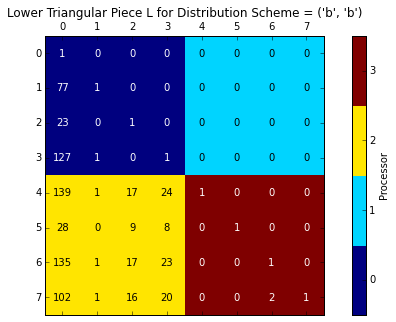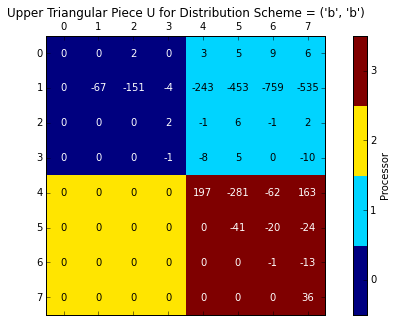Note: the values are not actually perfect integers, they are just displayed as such for the sake of readability.